RAOULT'S LAWHOME | C803-CFR | IN MEMORIAM | V. I. P. = V. I. P | SITE MAP | ALPHABETIC MAP | GLOBAL LAWS | ETHANOL | ATMOSPHERE | COPPER/PLASTIC | DISTILLATION | FEEDSTOCK\$ | FERMENTATION | BOILER / REBOILER | HEAT EXCHANGER | GO SOLAR | TOOLS | ELECTRO | ENGINES | S.E.E.F.A | LINKS | REFERENCES | APPENDICES | DOWNLOADS | ETHANOL FUEL MANUAL | FACTSRAOULT'S LAW AND NON-VOLATILE SOLUTES

This page deals with Raoult's Law and how it applies to solutions in which the solute is non-volatile - for example, a solution of salt in water. A non-volatile solute (the salt, for example) hasn't got any tendency to form a vapour at the temperature of the solution.

It goes on to explain how the resulting lowering of vapour pressure affects the boiling point and freezing point of the solution.

Important:  If you haven't already read the page about
saturated vapour pressure, you should follow this link before you go on.

Use the BACK button on your browser to return to this page when you are ready.

Raoult's Law

There are several ways of stating Raoult's Law, and you tend to use slightly different versions depending on the situation you are talking about. You can use the simplified definition in the box below in the case of a single volatile liquid (the solvent) and a non-volatile solute.

 The vapour pressure of a solution of a non-volatile solute is equal to the vapour pressure of the pure solvent at that temperature multiplied by its mole fraction.

In equation form, this reads:In this equation, Po is the vapour pressure of the pure solvent at a particular temperature.

xsolv is the mole fraction of the solvent. That is exactly what it says it is - the fraction of the total number of moles present which is solvent.

You calculate this using: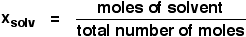Suppose you had a solution containing 10 moles of water and 0.1 moles of sugar. The total number of moles is therefore 10.1

The mole fraction of the water is: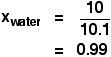A simple explanation of why Raoult's Law works

There are two ways of explaining why Raoult's Law works - a simple visual way, and a more sophisticated way based on entropy. Because of the level I am aiming at, I'm just going to look at the simple way.

Remember that saturated vapour pressure is what you get when a liquid is in a sealed container. An equilibrium is set up where the number of particles breaking away from the surface is exactly the same as the number sticking on to the surface again.Now suppose you added enough solute so that the solvent molecules only occupied 50% of the surface of the solution.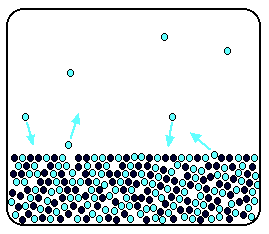A certain fraction of the solvent molecules will have enough energy to escape from the surface (say, 1 in 1000 or 1 in a million, or whatever). If you reduce the number of solvent molecules on the surface, you are going to reduce the number which can escape in any given time.

But it won't make any difference to the ability of molecules in the vapour to stick to the surface again. If a solvent molecule in the vapour hits a bit of surface occupied by the solute particles, it may well stick. There are obviously attractions between solvent and solute otherwise you wouldn't have a solution in the first place.

The net effect of this is that when equilibrium is established, there will be fewer solvent molecules in the vapour phase - it is less likely that they are going to break away, but there isn't any problem about them returning.

If there are fewer particles in the vapour at equilibrium, the saturated vapour pressure is lower.

Limitations on Raoult's Law

Raoult's Law only works for ideal solutions. An ideal solution is defined as one which obeys Raoult's Law.

Features of an ideal solution

In practice, there's no such thing! However, very dilute solutions obey Raoult's Law to a reasonable approximation. The solution in the last diagram wouldn't actually obey Raoult's Law - it is far too concentrated. I had to draw it that concentrated to make the point more clearly.

In an ideal solution, it takes exactly the same amount of energy for a solvent molecule to break away from the surface of the solution as it did in the pure solvent. The forces of attraction between solvent and solute are exactly the same as between the original solvent molecules - not a very likely event!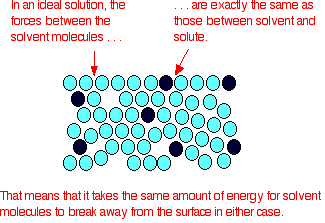Suppose that in the pure solvent, 1 in 1000 molecules had enough energy to overcome the intermolecular forces and break away from the surface in any given time. In an ideal solution, that would still be exactly the same proportion.

Fewer would, of course, break away because there are now fewer solvent molecules on the surface - but of those that are on the surface, the same proportion still break away.

If there were strong solvent-solute attractions, this proportion may be reduced to 1 in 2000, or 1 in 5000 or whatever.

In any real solution of, say, a salt in water, there are strong attractions between the water molecules and the ions. That would tend to slow down the loss of water molecules from the surface. However, if the solution is sufficiently dilute, there will be good-sized regions on the surface where you still have water molecules on their own. The solution will then approach ideal behaviour.

The nature of the solute

There is another thing that you have to be careful of if you are going to do any calculations on Raoult's Law (beyond the scope of this site). You may have noticed in the little calculation about mole fraction further up the page, that I used sugar as a solute rather than salt. There was a good reason for that!

What matters isn't actually the number of moles of substance that you put into the solution, but the number of moles of particles formed. For each mole of sodium chloride dissolved, you get 1 mole of sodium ions and 1 mole of chloride ions - in other words, you get twice the number of moles of particles as of original salt.So, if you added 0.1 moles of sodium chloride, there would actually be 0.2 moles of particles in the solution - and that's the figure you would have to use in the mole fraction calculation.

Unless you think carefully about it, Raoult's Law only works for solutes which don't change their nature when they dissolve. For example, they mustn't ionise or associate (in other words, if you put in substance A, it mustn't form A2 in solution).

If it does either of these things, you have to treat Raoult's law with great care.

Note:  This isn't a problem you are likely to have to worry about if you are a UK A level student. Just be aware that the problem exists.

Raoult's Law and melting and boiling points

The effect of Raoult's Law is that the saturated vapour pressure of a solution is going to be lower than that of the pure solvent at any particular temperature. That has important effects on the phase diagram of the solvent.

The next diagram shows the phase diagram for pure water in the region around its normal melting and boiling points. The 1 atmosphere line shows the conditions for measuring the normal melting and boiling points.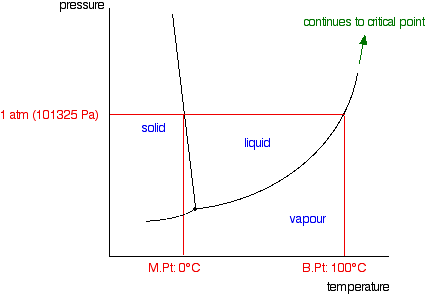Note:  In common with most phase diagrams, this is drawn highly distorted in order to show more clearly what is going on.

If you haven't already read my page about phase diagrams for pure substances, you should follow this link before you go on to make proper sense of what comes next.

Use the BACK button on your browser to return to this page when you are ready.

The line separating the liquid and vapour regions is the set of conditions where liquid and vapour are in equilibrium.

It can be thought of as the effect of pressure on the boiling point of the water, but it is also the curve showing the effect of temperature on the saturated vapour pressure of the water. These two ways of looking at the same line are discussed briefly in a note about half-way down the page about phase diagrams (follow the last link above).

If you draw the saturated vapour pressure curve for a solution of a non-volatile solute in water, it will always be lower than the curve for the pure water.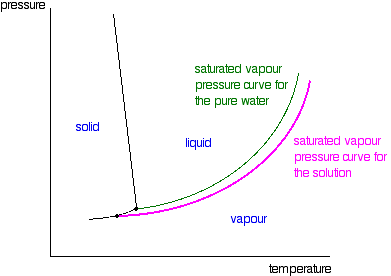Note:  The curves for the pure water and for the solution are often drawn parallel to each other. That has got to be wrong!

Suppose you have a solution where the mole fraction of the water is 0.99 and the vapour pressure of the pure water at that temperature is 100 kPa. The vapour pressure of the solution will be 99 kPa - a fall of 1 kPa. At a lower temperature, where the vapour pressure of the pure water is 10 kPa, the fall will only be 0.1 kPa. For the curves to be parallel the falls would have to be the same over the whole temperature range. They aren't!

If you look closely at the last diagram, you will see that the point at which the liquid-vapour equilibrium curve meets the solid-vapour curve has moved. That point is the triple point of the system - a unique set of temperature and pressure conditions at which it is possible to get solid, liquid and vapour all in equilibrium with each other at the same time.

Since the triple point has solid-liquid equilibrium present (amongst other equilibria), it is also a melting point of the system - although not the normal melting point because the pressure isn't 1 atmosphere.

That must mean that the phase diagram needs a new melting point line (a solid-liquid equilibrium line) passing through the new triple point. That is shown in the next diagram.Now we are finally in a position to see what effect a non-volatile solute has on the melting and freezing points of the solution. Look at what happens when you draw in the 1 atmosphere pressure line which lets you measure the melting and boiling points. The diagram also includes the melting and boiling points of the pure water from the original phase diagram for pure water (black lines).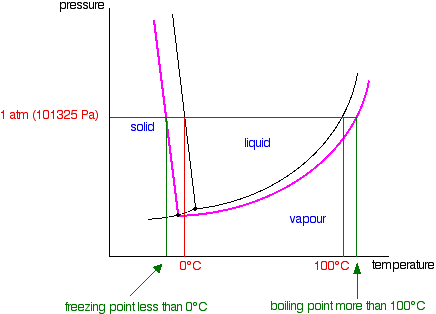Because of the changes to the phase diagram, you can see that:

• the boiling point of the solvent in a solution is higher than that of the pure solvent;

• the freezing point (melting point) of the solvent in a solution is lower than that of the pure solvent.

We have looked at this with water as the solvent, but using a different solvent would make no difference to the argument or the conclusions.

The only difference is in the slope of the solid-liquid equilibrium lines. For most solvents, these slope forwards whereas the water line slopes backwards. You could prove to yourself that that doesn't affect what we have been looking at by re-drawing all these diagrams with the slope of that particular line changed.

You will find it makes no difference whatsoever.

RAOULT'S LAW AND IDEAL MIXTURES OF LIQUIDS

This page deals with Raoult's Law and how it applies to mixtures of two volatile liquids. It covers cases where the two liquids are entirely miscible in all proportions to give a single liquid - NOT those where one liquid floats on top of the other (immiscible liquids).

The page explains what is meant by an ideal mixture and looks at how the phase diagram for such a mixture is built up and used.

Important:  If you haven't already read the page about saturated vapour pressure, you should follow this link before you go on.

Use the BACK button on your browser to return to this page when you are ready.

Ideal mixtures

An ideal mixture is one which obeys Raoult's Law, but I want to look at the characteristics of an ideal mixture before actually stating Raoult's Law. The page will flow better if I do it this way around.

Examples of ideal mixtures

There is actually no such thing as an ideal mixture! However, some liquid mixtures get fairly close to being ideal. These are mixtures of two very closely similar substances.

Commonly quoted examples include:

• hexane and heptane

• benzene and methylbenzene

• propan-1-ol and propan-2-ol

Ideal mixtures and intermolecular forces

In a pure liquid, some of the more energetic molecules have enough energy to overcome the intermolecular attractions and escape from the surface to form a vapour.

The smaller the intermolecular forces, the more molecules will be able to escape at any particular temperature.If you have a second liquid, the same thing is true. At any particular temperature a certain proportion of the molecules will have enough energy to leave the surface.In an ideal mixture of these two liquids, the tendency of the two different sorts of molecules to escape is unchanged.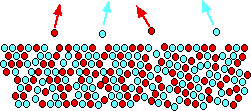You might think that the diagram shows only half as many of each molecule escaping - but the proportion of each escaping is still the same. The diagram is for a 50/50 mixture of the two liquids. That means that there are only half as many of each sort of molecule on the surface as in the pure liquids. If the proportion of each escaping stays the same, obviously only half as many will escape in any given time.

If the red molecules still have the same tendency to escape as before, that must mean that the intermolecular forces between two red molecules must be exactly the same as the intermolecular forces between a red and a blue molecule.

If the forces were any different, the tendency to escape would change.

Exactly the same thing is true of the forces between two blue molecules and the forces between a blue and a red. They must also be the same otherwise the blue ones would have a different tendency to escape than before.

If you follow the logic of this through, the intermolecular attractions between two red molecules, two blue molecules or a red and a blue molecule must all be exactly the same if the mixture is to be ideal.

This is why mixtures like hexane and heptane get close to ideal behaviour. They are similarly sized molecules and so have similarly sized van der Waals attractions between them. However, they obviously aren't identical - and so although they get close to being ideal, they aren't actually ideal.

For the purposes of this topic, getting close to ideal is good enough!

Ideal mixtures and enthalpy change of mixing

When you make any mixture of liquids, you have to break the existing intermolecular attractions (which needs energy), and then remake new ones (which releases energy).

If all these attractions are the same, there won't be any heat either evolved or absorbed.

That means that an ideal mixture of two liquids will have zero enthalpy change of mixing. If the temperature rises or falls when you mix the two liquids, then the mixture isn't ideal.

Raoult's Law

You may have come cross a slightly simplified version of Raoult's Law if you have studied the effect of a non-volatile solute like salt on the vapour pressure of solvents like water. The definition below is the one to use if you are talking about mixtures of two volatile liquids.

 The partial vapour pressure of a component in a mixture is equal to the vapour pressure of the pure component at that temperature multiplied by its mole fraction in the mixture.

Raoult's Law only works for ideal mixtures.

In equation form, for a mixture of liquids A and B, this reads: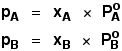In this equation, PA and PB are the partial vapour pressures of the components A and B. In any mixture of gases, each gas exerts its own pressure. This is called its partial pressure and is independent of the other gases present. Even if you took all the other gases away, the remaining gas would still be exerting its own partial pressure.

The total vapour pressure of the mixture is equal to the sum of the individual partial pressures.The Po values are the vapour pressures of A and B if they were on their own as pure liquids.

xA and xB are the mole fractions of A and B. That is exactly what it says it is - the fraction of the total number of moles present which is A or B.

You calculate mole fraction using, for example:Putting all this together in a simple example:

Suppose you had a mixture of 2 moles of methanol and 1 mole of ethanol at a particular temperature. The vapour pressure of pure methanol at this temperature is 81 kPa, and the vapour pressure of pure ethanol is 45 kPa.

There are 3 moles in the mixture in total.

2 of these are methanol. The mole fraction of methanol is 2/3.

Similarly, the mole fraction of ethanol is 1/3.

You can easily find the partial vapour pressures using Raoult's Law - assuming that a mixture of methanol and ethanol is ideal.

First for methanol:. . . and then for ethanol: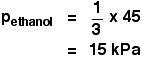You get the total vapour pressure of the liquid mixture by adding these together.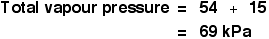In practice, this is all a lot easier than it looks when you first meet the definition of Raoult's Law and the equations!

Vapour pressure / composition diagrams

Suppose you have an ideal mixture of two liquids A and B. Each of A and B is making its own contribution to the overall vapour pressure of the mixture - as we've seen above.

Let's focus on one of these liquids - A, for example.

Suppose you double the mole fraction of A in the mixture (keeping the temperature constant). According to Raoult's Law, you will double its partial vapour pressure. If you triple the mole fraction, its partial vapour pressure will triple - and so on.

In other words, the partial vapour pressure of A at a particular temperature is proportional to its mole fraction. If you plot a graph of the partial vapour pressure of A against its mole fraction, you will get a straight line.Now we'll do the same thing for B - except that we will plot it on the same set of axes. The mole fraction of B falls as A increases so the line will slope down rather than up. As the mole fraction of B falls, its vapour pressure will fall at the same rate.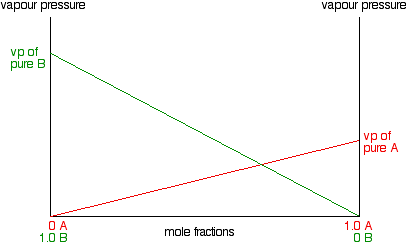Notice that the vapour pressure of pure B is higher than that of pure A. That means that molecules must break away more easily from the surface of B than of A. B is the more volatile liquid.

Note:  Two things. First, because the intermolecular forces in the two liquids aren't exactly the same, they aren't going to form a strictly ideal mixture. However, if we make them identical, it would turn out that everything else we say in this topic would be completely pointless!

Secondly, the choice of which of these liquids is the more volatile is totally arbitrary. I could equally well have drawn a different diagram where A was the more volatile and had the higher vapour pressure. I could also have reversed the mole fraction scale with pure A on the left-hand side and pure B on the right. All of this, of course, will mean that these diagrams (and all those that follow) could look subtly different if you find them from different sources. It is really important with this to understand what is going on, otherwise you risk getting seriously confused.

To get the total vapour pressure of the mixture, you need to add the values for A and B together at each composition. The net effect of that is to give you a straight line as shown in the next diagram.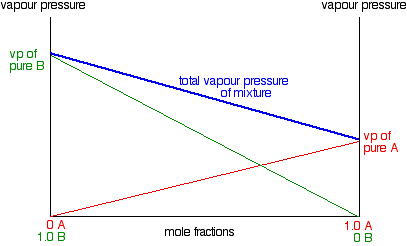Following on from the last note:  For non-ideal mixtures, these straight lines become curves. For a nearly ideal mixture, they are near enough straight lines - that's the assumption we are working on here. The less ideal the mixture is, the more curved the lines become. This is dealt with in more detail on another page. I'm not giving you a link to that at the moment, because you shouldn't visit that page until you've finished this one - it would be too scary!

Boliling point / composition diagrams

The relationship between boiling point and vapour pressure

If a liquid has a high vapour pressure at a particular temperature, it means that its molecules are escaping easily from the surface.

If, at the same temperature, a second liquid has a low vapour pressure, it means that its molecules aren't escaping so easily.

What does that imply about the boiling points of the two liquids? Don't read on until you have tried to think this out!

There are two ways of looking at this. Choose whichever seems easiest to you. It doesn't matter how you work this out - it is the result that is important.

Either:

• If the molecules are escaping easily from the surface, it must mean that the intermolecular forces are relatively weak. That means that you won't have to supply so much heat to break them completely and boil the liquid.

The liquid with the higher vapour pressure at a particular temperature is the one with the lower boiling point.

Or:

• Liquids boil when their vapour pressure becomes equal to the external pressure. If a liquid has a high vapour pressure at some temperature, you won't have to increase the temperature very much until the vapour pressure reaches the external pressure. On the other hand if the vapour pressure is low, you will have to heat it up a lot more to reach the external pressure.

The liquid with the higher vapour pressure at a particular temperature is the one with the lower boiling point.

Don't go on until you are sure about this:

For two liquids at the same temperature:

• The one with the higher vapour pressure is the one with the lower boiling point.

Constructing a boiling point / composition diagram

To remind you - we've just ended up with this vapour pressure / composition diagram:We're going to convert this into a boiling point / composition diagram.

We'll start with the boiling points of pure A and B.

B has the higher vapour pressure. That means that it will have the lower boiling point. If that isn't obvious to you, go back and read the last section again!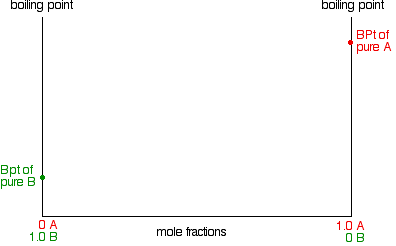For mixtures of A and B, you might perhaps have expected that their boiling points would form a straight line joining the two points we've already got. Not so!

In fact, it turns out to be a curve.Important:  Take great care drawing this curve. The boiling point of B is the lowest boiling point. Don't let your curve droop below this. That happens with certain non-ideal mixtures and has consequences which are explored on another page.

To make this diagram really useful (and finally get to the phase diagram we've been heading towards), we are going to add another line. This second line will show the composition of the vapour over the top of any particular boiling liquid.

If you boil a liquid mixture, you would expect to find that the more volatile substance escapes to form a vapour more easily than the less volatile one.

That means that in the case we've been talking about, you would expect to find a higher proportion of B (the more volatile component) in the vapour than in the liquid. You can discover this composition by condensing the vapour and analysing it. That would give you a point on the diagram.The diagram just shows what happens if you boil a particular mixture of A and B. Notice that the vapour over the top of the boiling liquid has a composition which is much richer in B - the more volatile component.

If you repeat this exercise with liquid mixtures of lots of different compositions, you can plot a second curve - a vapour composition line.This is now our final phase diagram.

Important:  Once again, take great care drawing this second curve. No point on the curve must be higher than the boiling temperature of the pure A.

Using the phase diagram

The diagram is used in exactly the same way as it was built up. If you boil a liquid mixture, you can find out the temperature it boils at, and the composition of the vapour over the boiling liquid.

For example, in the next diagram, if you boil a liquid mixture C1, it will boil at a temperature T1 and the vapour over the top of the boiling liquid will have the composition C2.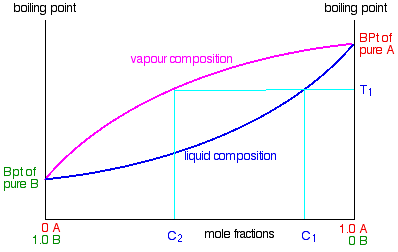All you have to do is to use the liquid composition curve to find the boiling point of the liquid, and then look at what the vapour composition would be at that temperature.

Notice again that the vapour is much richer in the more volatile component B than the original liquid mixture was.

The beginnings of fractional distillation

Suppose that you collected and condensed the vapour over the top of the boiling liquid and reboiled it.

You would now be boiling a new liquid which had a composition C2.

That would boil at a new temperature T2, and the vapour over the top of it would have a composition C3.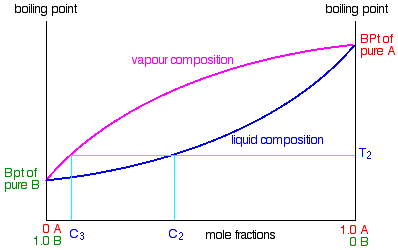You can see that we now have a vapour which is getting quite close to being pure B. If you keep on doing this (condensing the vapour, and then reboiling the liquid produced) you will eventually get pure B.

This is obvious the basis for fractional distillation. However, doing it like this would be incredibly tedious, and unless you could arrange to produce and condense huge amounts of vapour over the top of the boiling liquid, the amount of B which you would get at the end would be very small.

Real fractionating columns (whether in the lab or in industry) automate this condensing and reboiling process. How these work will be explored on another page (see below).

`                  `Enter supporting content here